# Project 8 - Tessellation

In this project we will implement simple normal mapping and displacement mapping. This project will include using both tessellation shaders and geometry shaders.

Requirements

• Step 1: Normal mapping
• Your program must take the name of a .png image file as its first command-line argument.
• This image will be used as the normal map texture.
• You can use this image for your tests.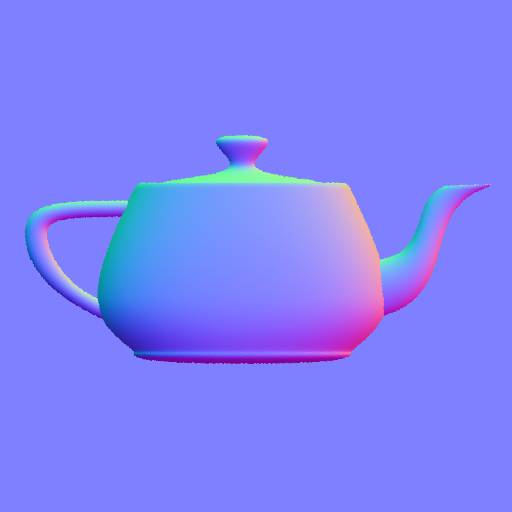An example normal map image.
• Use the normal map texture in the fragment shader to set the surface normal.
• Use Blinn shading with non-zero diffuse and specular components.An example plane with a normal mapping.
• Step 2: Show triangulation
• The plane should have only two triangles.
• Write a geometry shader that take a triangle and convert it into line segments, showing the edges of the triangle.
• Use this geometry shader to draw the triangle edges, showing the triangulation.
• Note that you should first draw the plane as in step 1 and then draw it again with this geometry shader.
• Make sure to move the line segments slightly closer to the camera, so that they are not occluded by the plane.
• The space key should control whether the triangulation is displayed.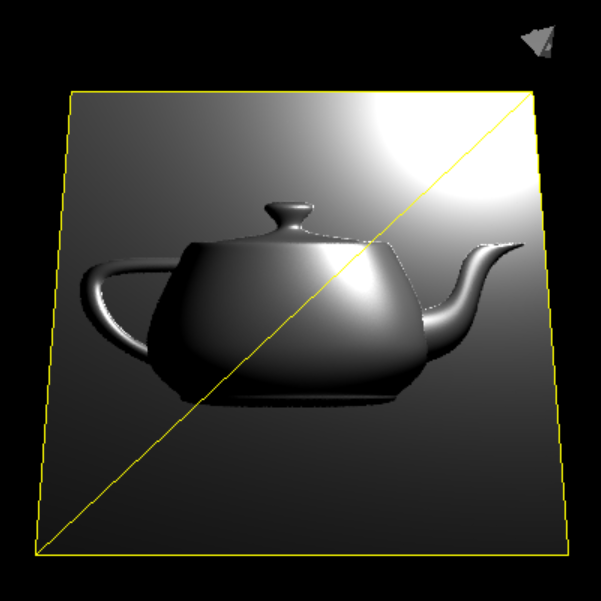An example plane with a normal map, showing triangulation.
• Step 3: Displacement mapping
• Your program must take the name of a second .png image file as its second command-line argument.
• This second image will be used as the displacement map.
• If there is no second command-line argument, your program should NOT use displacement mapping and should only show the results of Steps 1 and 2.
• You can use this image for your tests.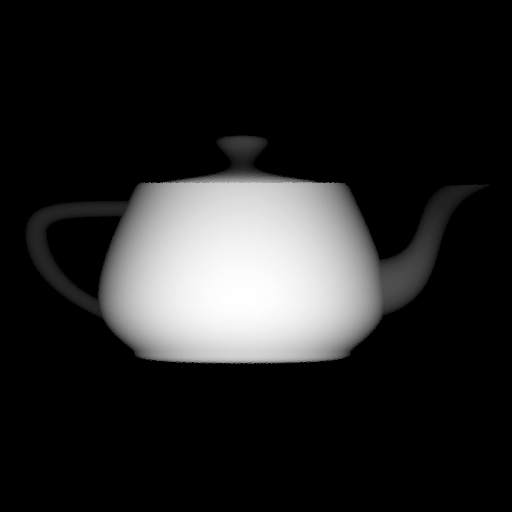An example displacement map image.
• Write a tessellation shader that takes the plane from Steps 1 and 2 and tessellates it into many triangles.
• Left and right arrow keys should control the tessellation level.
• Move the vertices of the tessellated plane up using the displacement map values in the tessellation evaluation shader.
• Note that when using displacement mapping, you might want to handle the transformations in the tessellation evaluation shader, instead of the vertex shader.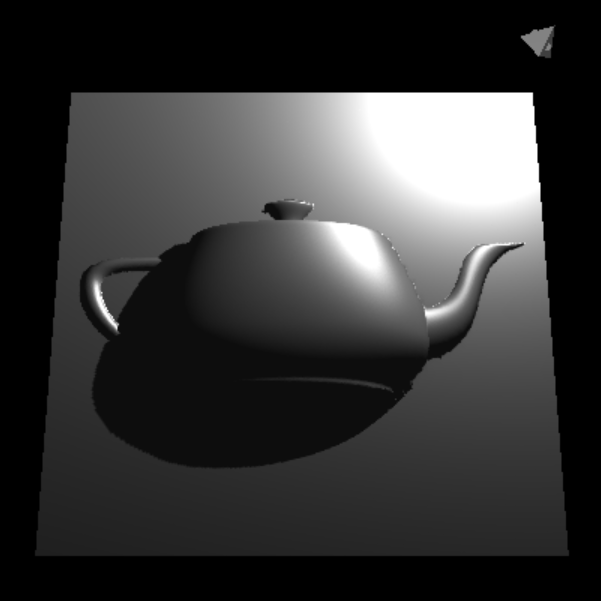An example plane with a displacement map.
• Use the same or a similar geometry shader as in Step 2 to display the triangulation.
• As above, the space key should control whether the triangulation is displayed.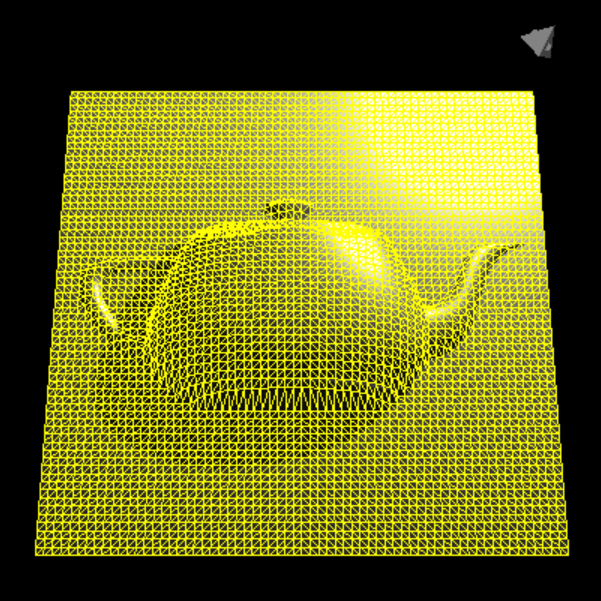An example plane with a displacement map, showing triangulation.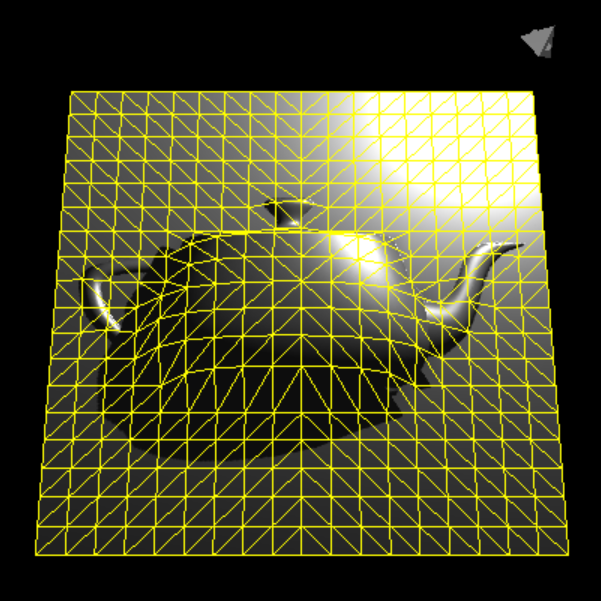An example plane with a displacement map, showing triangulation of a lower tessellation level.

Additional Requirements for CS 6610 Students